Perimeter

Chapter 10 Class 6 Mensuration
Concept wise

Suppose we have a park like this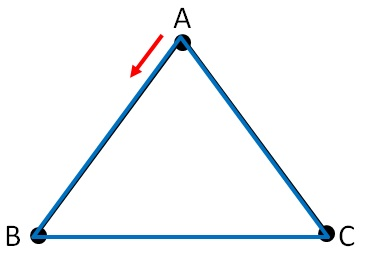And I run along the boundary from point A & back to point A

This blue line is the perimeter of the figure

Perimeter is distance along the boundary of a closed figure

Let’s take some examples.

#### Find Perimeter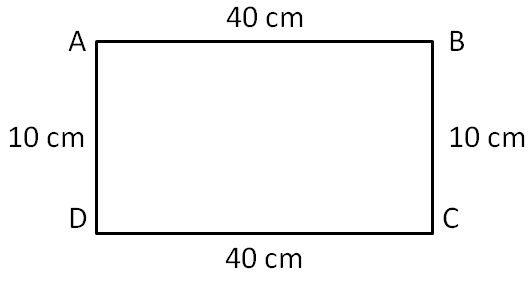Perimeter = Sum of all sides

= AB + BC + CD + AD

= 40 + 10 + 40 + 10

= 100 cm

#### Find Perimeter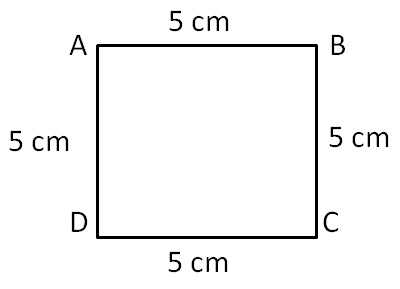Perimeter = Sum of all sides

= AB + BC + CD + DA

= 5 + 5 + 5 + 5

= 20 cm

#### Find Perimeter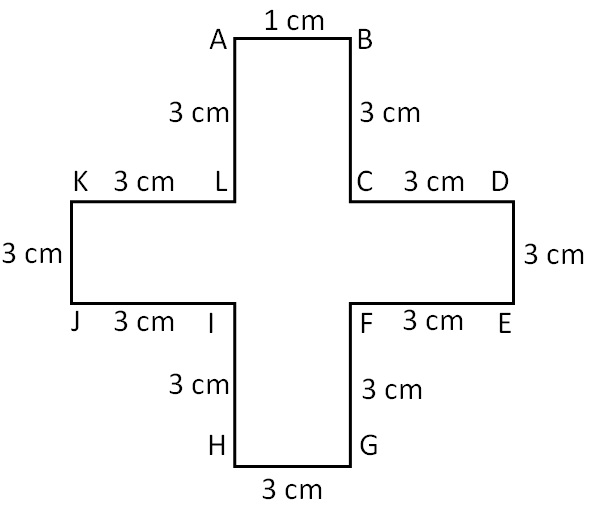Perimeter = LA + AB + BC

+ CD + DE + EF

+ FG + GH + HI

+ IJ + JK + KL

= 3 + 1 + 3 + 3 + 1 + 3 + 3 + 1 + 3 + 3 + 1 + 3

= 7 + 7 + 7 + 7

= 28 cm

#### Find Perimeter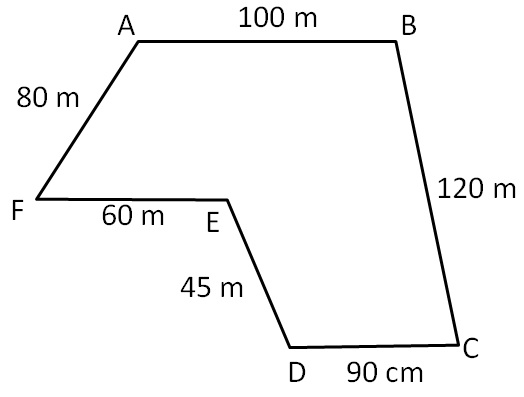Perimeter = AB + BC + CD + DE + EF + FA

= 100 + 120 + 90 + 45 + 60 + 80

= 220 + 135 + 140

= 355 + 140

= 495 m

Learn in your speed, with individual attention - Teachoo Maths 1-on-1 Class